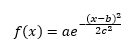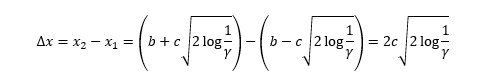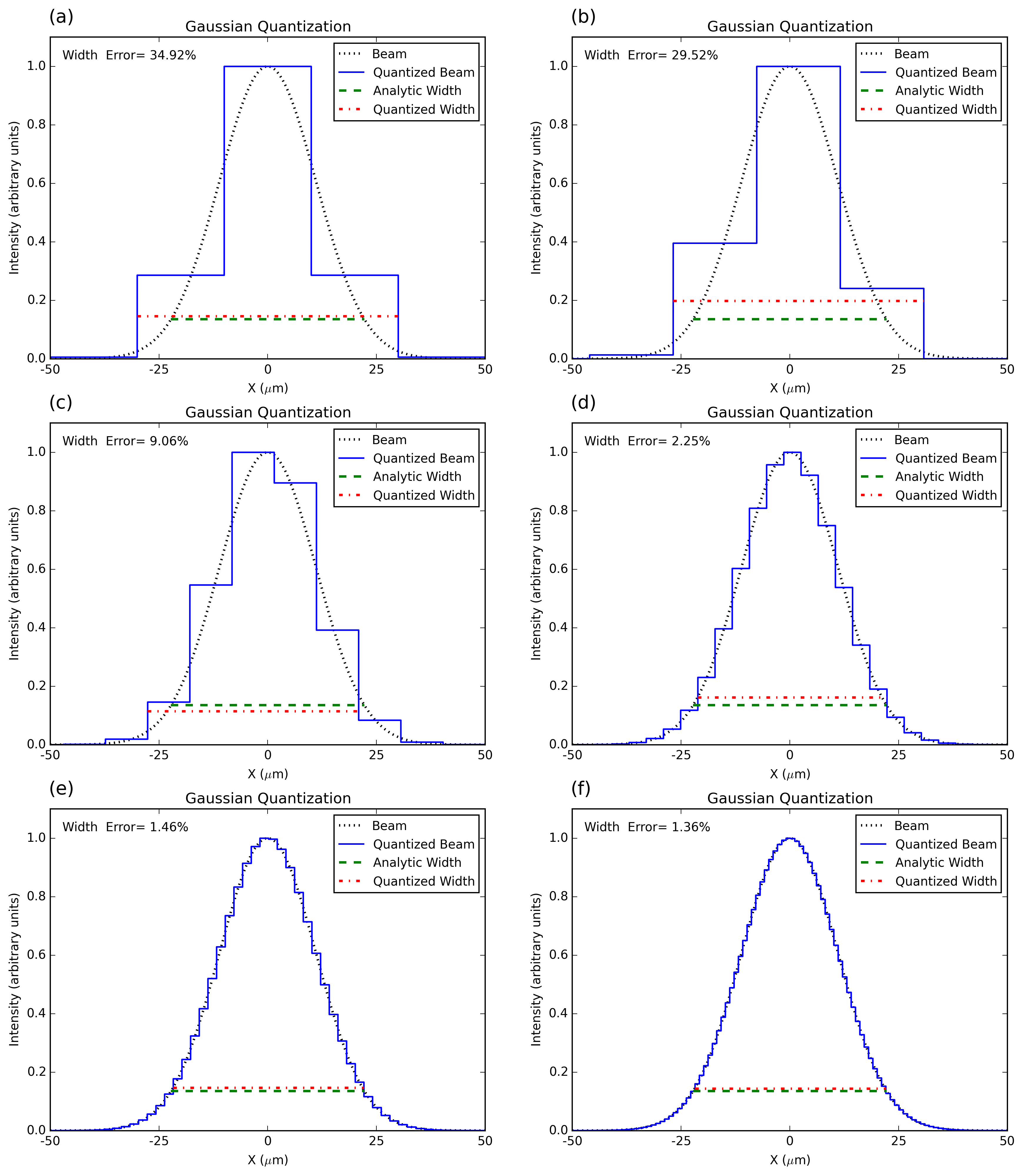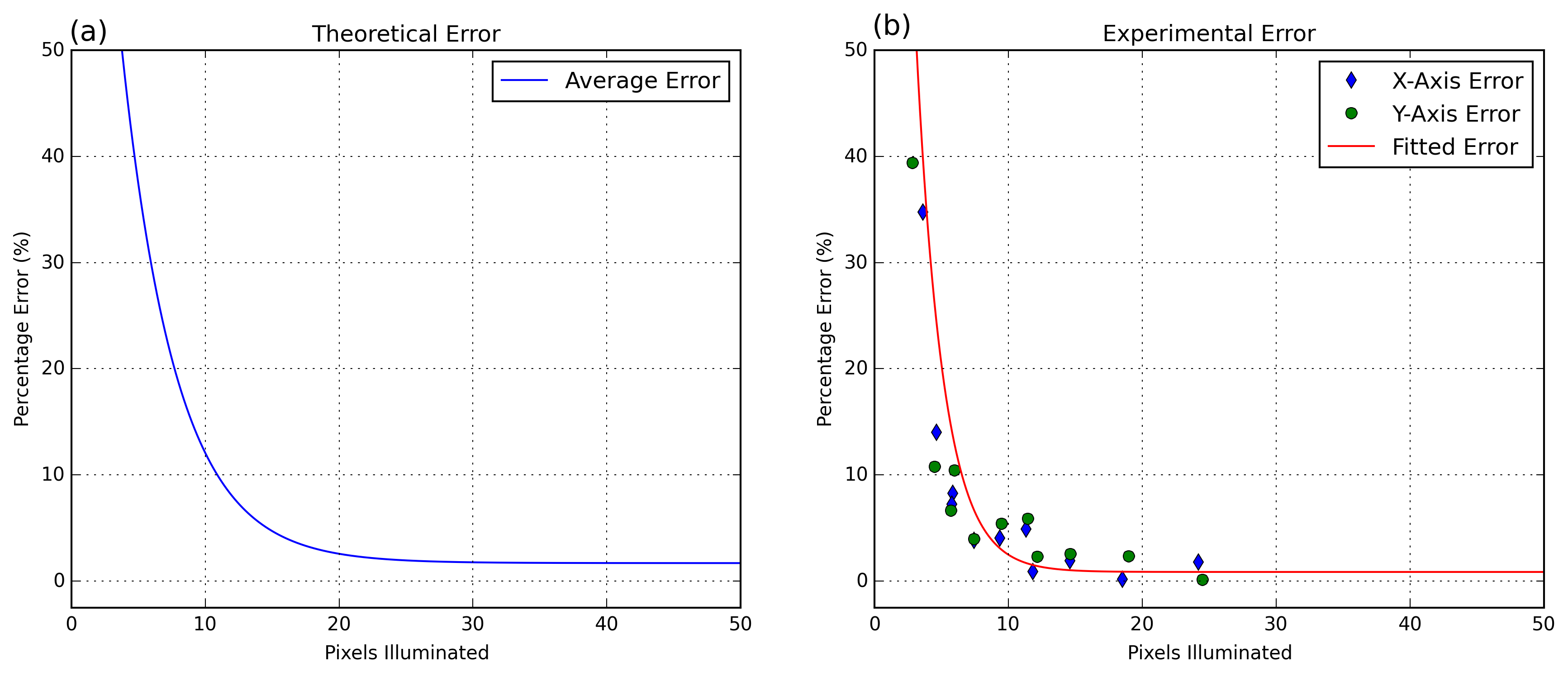March 14, 2016

# Small Beam Width Theoretical and Experimental Error

One of the most important measurements in laser beam profiling is the beam width measurement. A common question we see is “How small of a beam can I measure with this camera?” Generally, we recommend that the user ensures that they cover at least 10 camera pixels along each axis to get a good measurement. The rest of this blog post will examine why we provide this guideline, discuss the theory behind it, and use some measured data gathered from several DataRay cameras to validate the theory. We will also provide what approximate error values to expect when covering small numbers of pixels.

With two dimensional sensors such as a CCD or CMOS, advanced beam width algorithms can be used (see ISO 11146 Standard); however, to simplify the discussion, we will use a simpler method using only the beam profile along an axis. A beam striking a sensor illuminates a certain number of pixels. The ADC values of the illuminated pixels are proportional to the intensity and power (we assume the intensity is constant across the pixel and thus the power is a scalar multiple of the intensity) and thus we can plot the intensity against the pixel position to obtain a profile of the beam. The simplest way of determining the width from the beam profile is the clip percentage method. First, the maximum intensity (α) of the beam profile is determined and a certain percentage cutoff (y) is set (we use 13.5%). An algorithm searches to find where the beam intensity is equal to α and returns the pixel coordinates. For almost all beams, there are two such pixel coordinates and by determining the distance between them the beam width can be found.

The error in the width of the beam being profiled is directly proportional to the number of pixels that are illuminated. With an infinite number of illuminated pixels (requiring infinitely small pixels), the true profile of the beam would be represented. However, CCD and CMOS sensors have finite pixel dimensions which cause a discretization of the returned beam profile. The discretization causes errors in the beam width measurement; therefore, the beam must illuminate a minimum number of pixels for an accurate beam width measurement. To determine the minimum amount of pixels that must be illuminated, a theoretical model was created and tested against experimental data.

#### Theoretical model

First, the profile of a perfect Gaussian beam was generated to represent the profile of a beam incident on a sensor (see Figure 1). The beam width can be analytically determined by using the Gaussian formulaWhere a, b and c are all constants. By setting f(x) = γa, where 0 ≤ γ ≤ 1, and then solving for the x values, the beam’s width is found to beTo approximate how the sensor reads the beam intensity, a number of equally sized bins m along the x-axis were created. A bin represents one pixel; therefore, m represents the number of pixels illuminated. The beam intensity was then integrated across the width of each bin and the values normalized by setting the maximum bin value equal to the maximum of the Gaussian beam. The bins were then plotted alongside the Gaussian. After quantizing the Gaussian, the quantized beam’s width was determined. The percentage error was calculated by comparing the quantized beam’s width against the analytically determined width (see Figure 1).Figure 1: (a) The Gaussian beam (dotted) is approximated by the quantized beam (solid). The quantized beam has =5. The analytic width (dashed) is compared to the quantized width (dash-dot) to give the Width Percentage error listed. Note the quantized beam is symmetric. (b) Although is still equal to 5, by changing the alignment of the beam on the pixels, the quantized beam becomes asymmetric. (c) =10. (d) =25. (e) =50. (f) =100. Note that as the number of pixels illuminated grows larger, the quantized beam better approximates the Gaussian and the error decreases.

We experimented with different values of m, from m=5 to m=100. As the number of pixels illuminated increased, the quantized beam provided a better approximation of the Gaussian, and the average error of the quantized beam’s width measurement decreased. We also randomly offset the Gaussian beam such that the center of the Gaussian did not always fall in the exact center of a pixel. If the beam is perfectly aligned on the pixels, then a symmetric quantization will be seen (see Figure 1a). However, if the beam is shifted slightly, then an asymmetric quantization will be seen (see Figure 1b). A variety of different alignments for each m value were simulated and the percentage errors recorded (see Figure 1c-Figure 1f). The error for different alignments at the various levels m were averaged to provide a better estimation of the true error. Finally, the percentage errors were fitted to a decaying exponential curve (see Figure 2a).Figure 2: (a) Percentage error as a function of the pixels illuminated. The average error (solid) for the theoretical error is shown as a decaying exponential curve. (b) The x-axis error (diamond) and the y-axis error (circle) are shown. To provide comparison against the theoretical data, a decaying exponential curve is fitted through the data.

#### Experiment

To prove the validity of our theoretical model, we devised an experiment to find the percentage error vs. pixels illuminated. The beam waist of a focused 675 nm Gaussian beam was measured with a number of cameras and sensors. The Beam'R2, is one of our scanning slit devices and has a resolution of 0.1µm which is at least 32 times smaller than the CCD or CMOS camera resolution, the Beam'R2 was used to determine the correct beam width. The beam width was then measured with three other beam profilers: the BladeCam-XHR, the TaperCamD20-15-UCD23 and finally, our flagship profiler, the WinCamD-LCM4. Using these three cameras with both full and fast mode (which effectively doubles the pixel size), we were able to achieve six different pixel sizes. Since we generated two different beam widths by using two lenses, a total of twelve different measurements were made. Furthermore, each measurement included an x and y axis for a total of twenty-four data points. The measured width of the beam was divided by the pixel size to give the number of pixels illuminated. The percentage error of the beam width was calculated by comparison with the Beam'R2 control width. Finally, the percentage error vs. the pixels illuminated was plotted with a decaying exponential curve fitted through the data. The experimental results followed the theoretical results and we see similar decaying exponential curves in both the experimental and theoretical data.

#### Conclusion

DataRay states that a minimum of ten pixels should be illuminated for a beam width measurement. From the theoretical model, ten illuminated pixels corresponds to approximately a 10% error, while the experimental data shows that a 5% error was achieved. For more information on measuring small beams and the best software settings to use, please see our previous blog post.

Laser beam profiling cameras and scanning slit detectors well suited to any application can be found on our website. Should you have any further laser beam profiling or pixel accuracy questions, feel free to contact us.

Posted by: Lucas Hofer